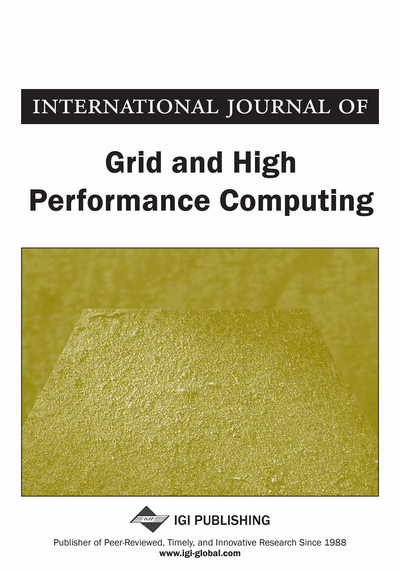# Rhotrix Multiplication on Two-Dimensional Process Grid Topologies

Ezugwu E. Absalom (Ahmadu Bello University, Zaria, Nigeria) and Junaidu B. Sahalu (Ahmadu Bello University, Zaria, Nigeria)
DOI: 10.4018/jghpc.2012010102
Available
\$37.50
No Current Special Offers

## Abstract

This paper presents rhotrix multiplication and introduces its implementation on process grid topologies. Covered in this work, are reviews of generalization cases of n-dimensional rhotrix multiplication. A parallel program based on MPI master-worker paradigm is implemented. By means of analyzing the time complexity and memory requirement for the method, the authors provided insight into how to fully utilize the algorithm for heart-oriented rhotrix multiplication in anticipation of establishing a standard for parallel rhotrix multiplication and to speed up its multiplication based on existing HPC tools.
Article Preview
Top

## Introduction

Rhotrix is a mathematical concept introduced in Ajibade (2003) as an extension of the initiative formerly suggested by Atanassove and Shannon (1998). The algebraic structures and analysis of rhotrices was presented in Ajibade (2003) and alternative approach given in relation to matrix algebra is presented in Sani (2004).

Where a, b, d, e, h(R) ∊ ℝ, and h(R) is called the heart of a rhotrix R.

The multiplication of rhotrices as defined by Ajibade (2003) is given as follows: Assuming that R and Q are two rhotrices, then ifwe have that

Where “+” and “” defines addition and multiplication over R and Q, respectively, while h(R) and h(Q) are the respective hearts of the two rhotrices.

In this paper we present a parallel implementation of heart-oriented rhotrix multiplication over a tow-dimensional grid topology by adopting the master-worker paradigm. The formal representation for heart-oriented multiplication of n-dimensional rhotrices was presented in Ezugwu et al. (2011). This gave room for the initial conception of the parallel computation upon which this paper is bases.

Top

## Rhotrix Multiplication

An expression for the resultant multiplication of two n-dimensional rhotrices Rn and Qn, which was introduced in Ezugwu et al. (2011), is expressed as shown in expression 1.

Expression 1
``

The mathematical model is interpreted as follows:

The entries of the product rhotrix Cn, of Rn and Qn is expressed as follows:, for i = 1,2,…,(2) where λ = 0 when the index value corresponds to that of the heart and λ = 1 when otherwise.

We can also represent (1) in a two-dimension view as introduced in Ezugwu et al. (2011), which is denoted as show in expression 2.

Expression 2
``

The mathematical model is expressed as follows:

(4)

## Complete Article List

Search this Journal:
Reset
Open Access Articles: Forthcoming
Volume 14: 6 Issues (2022): Forthcoming, Available for Pre-Order
Volume 13: 4 Issues (2021)
Volume 12: 4 Issues (2020)
Volume 11: 4 Issues (2019)
Volume 10: 4 Issues (2018)
Volume 9: 4 Issues (2017)
Volume 8: 4 Issues (2016)
Volume 7: 4 Issues (2015)
Volume 6: 4 Issues (2014)
Volume 5: 4 Issues (2013)
Volume 4: 4 Issues (2012)
Volume 3: 4 Issues (2011)
Volume 2: 4 Issues (2010)
Volume 1: 4 Issues (2009)
View Complete Journal Contents Listing